# 降维和特征选择的关键方法介绍及MATLAB实现

MATLAB实现

【例】光谱数据主成分回归分析（PCR）

【例】偏最小二乘法（PLS）

Filter vs. Wrapper

# 降维的方法

## 主成分分析（Principle Component Analysis, PCA）方法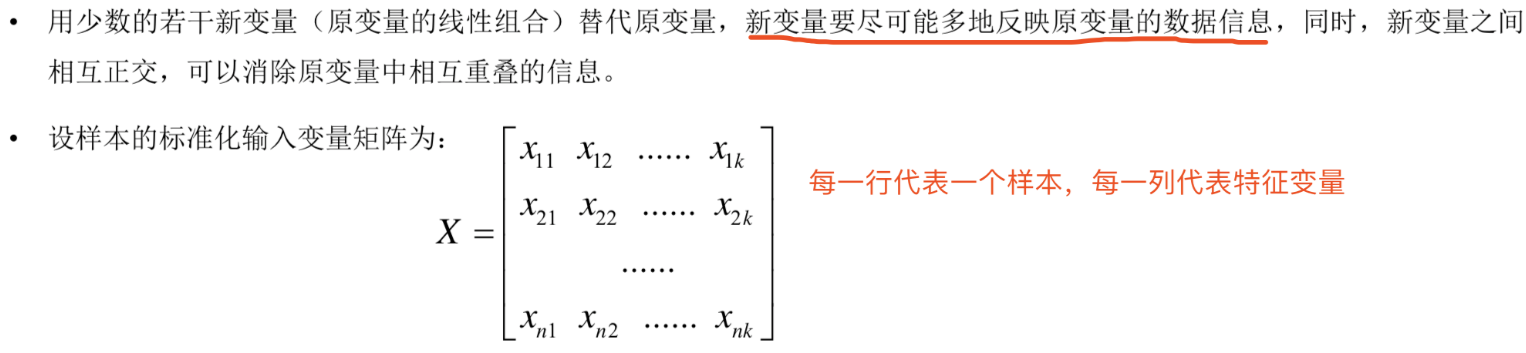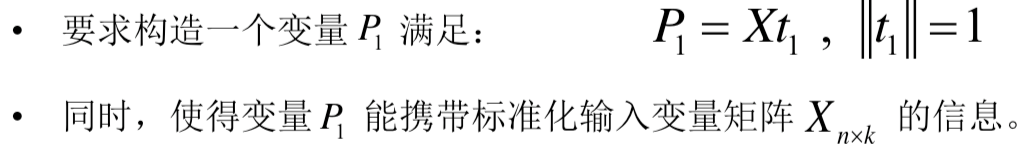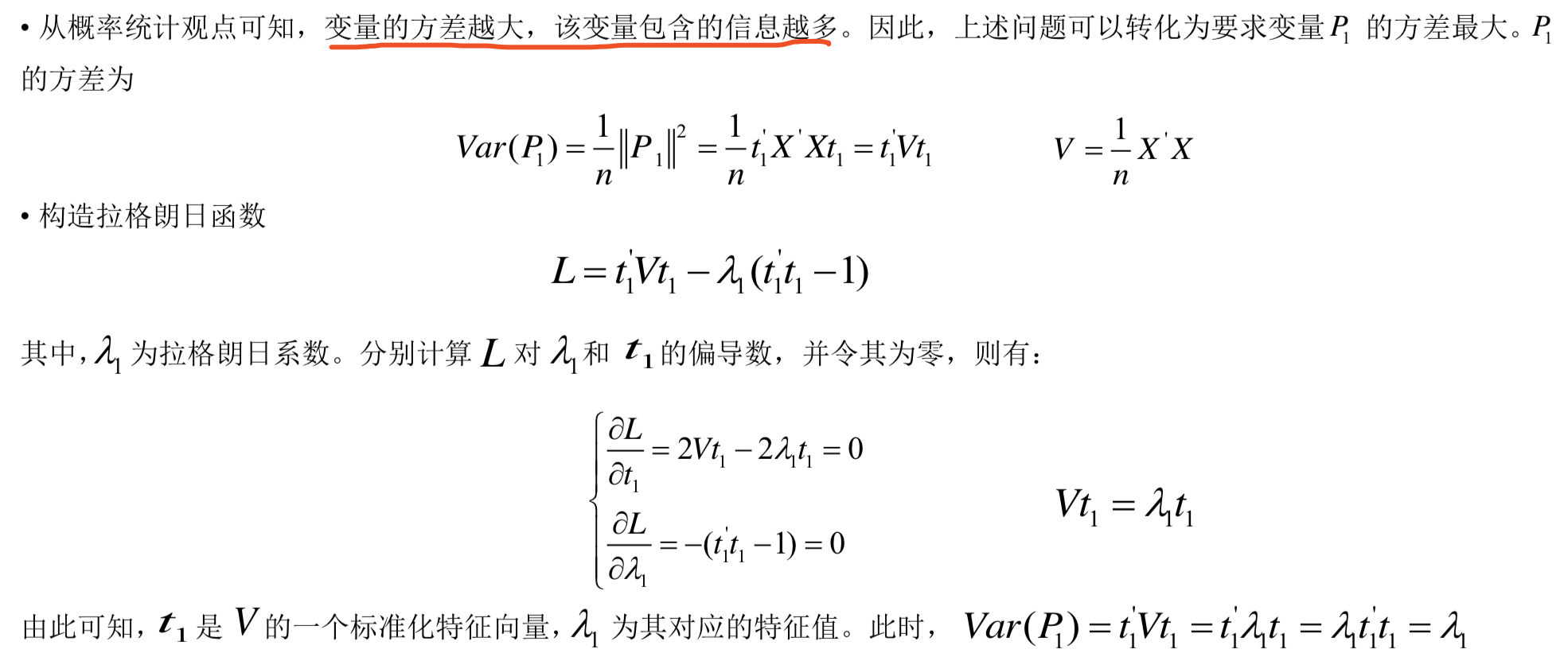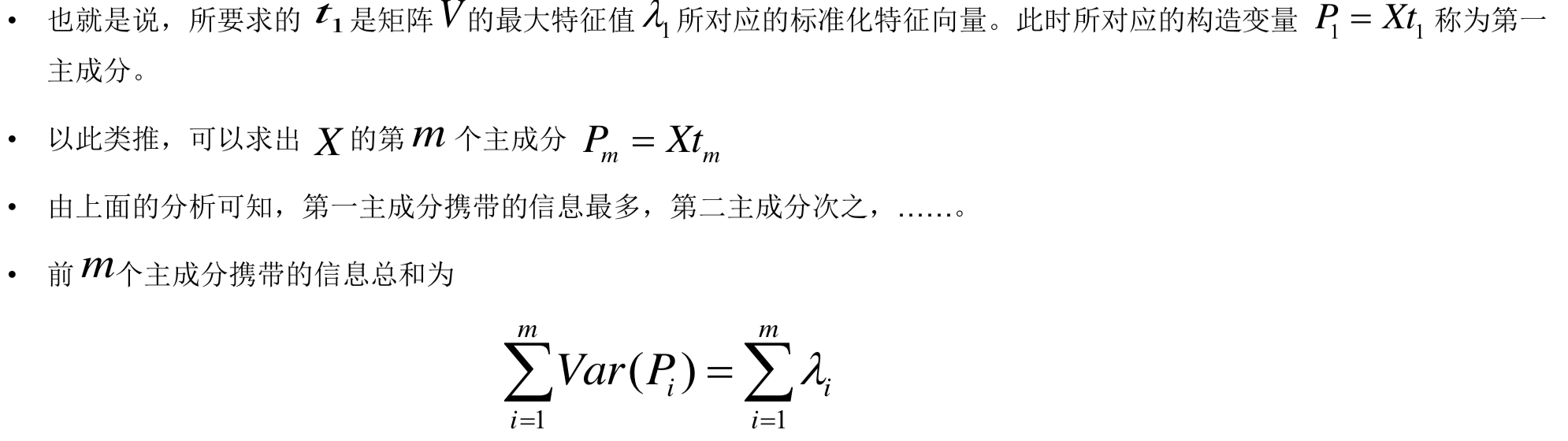## 偏最小二乘法(Partial Least Squares, PLS)

• PCA方法提取出的前若干个主成分携带了原输入变量矩阵的大部分信息，消除了相互重叠部分的信息。但没有考虑主成分对输出变量的解释能力方差贡献率很小但对输出变量有很强解释能力的主成分将会被忽略掉，这无疑会对校正模型的性能产生一定的影响。偏最小二乘法(PLS)可以很好地解决这个问题。

• PLS的基本思路是逐步回归，逐步分解输入变量矩阵和输出变量矩阵，并综合考虑提取的主成分对输入变量矩阵和输出变量矩阵的解释能力，直到满足性能要求为止。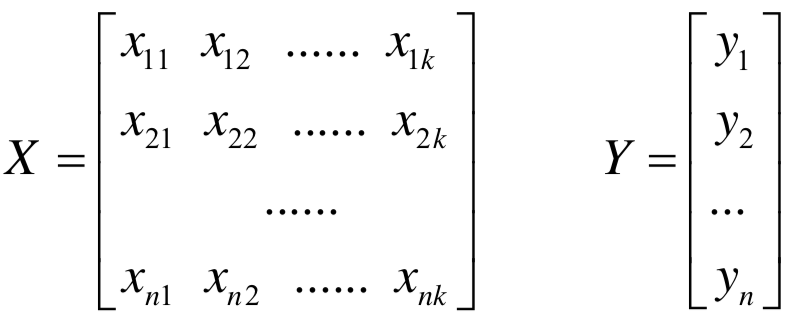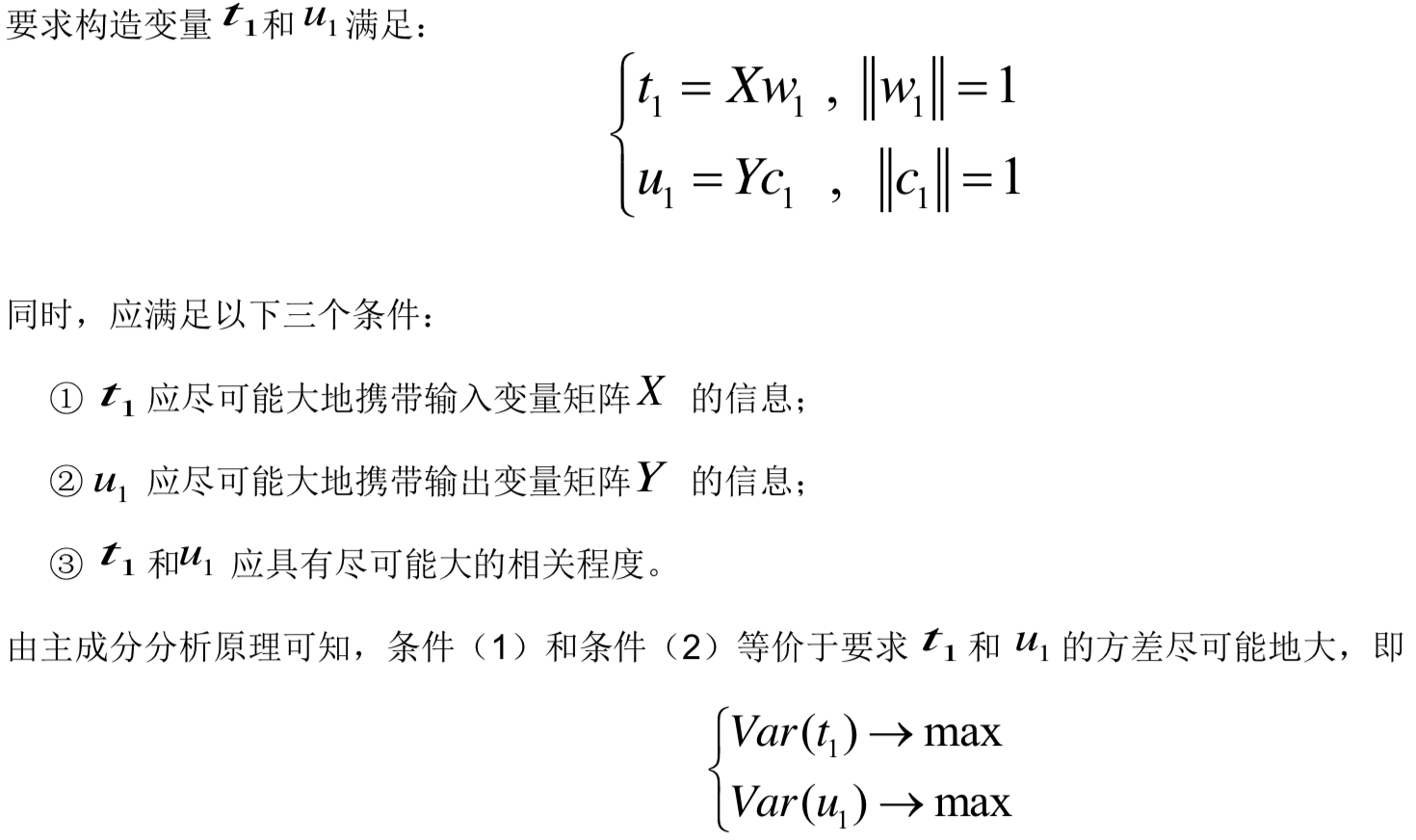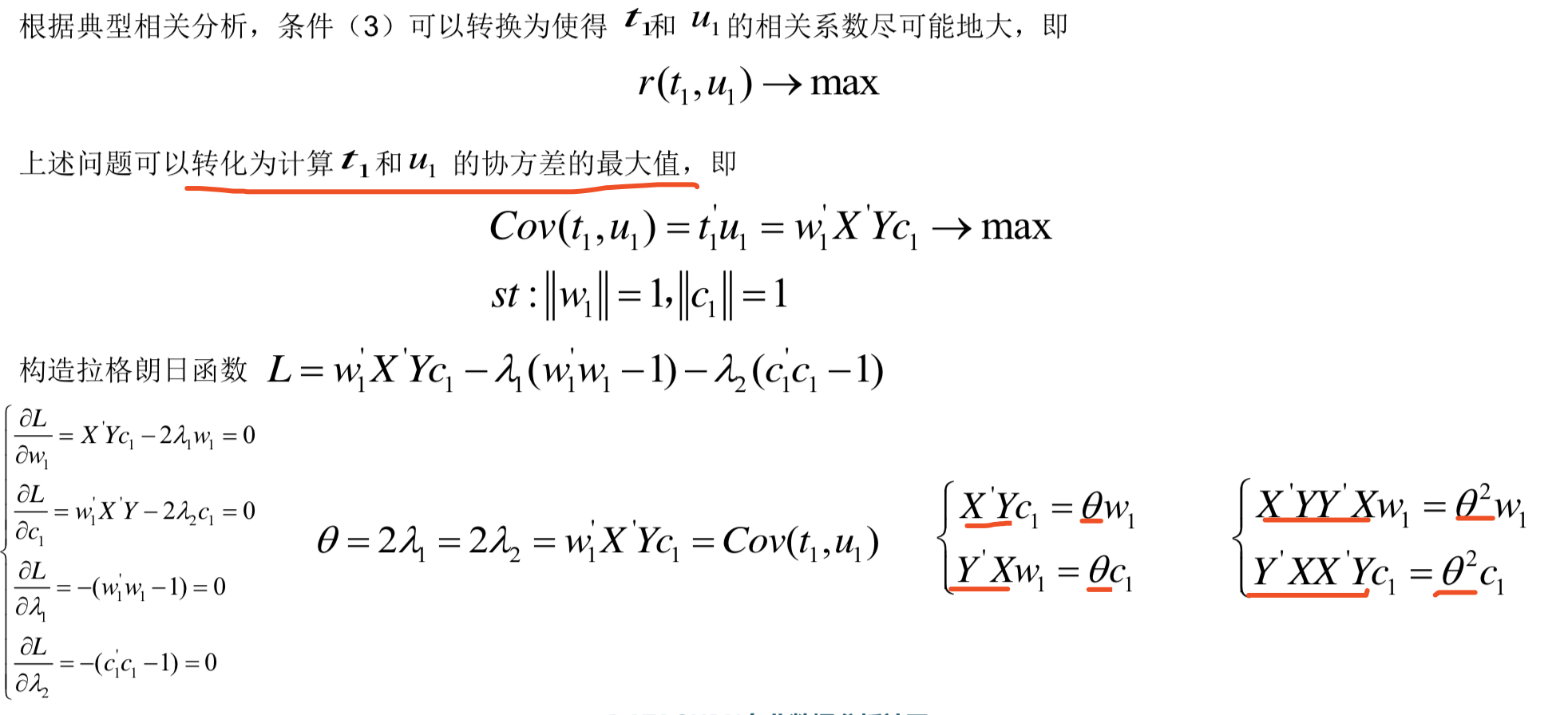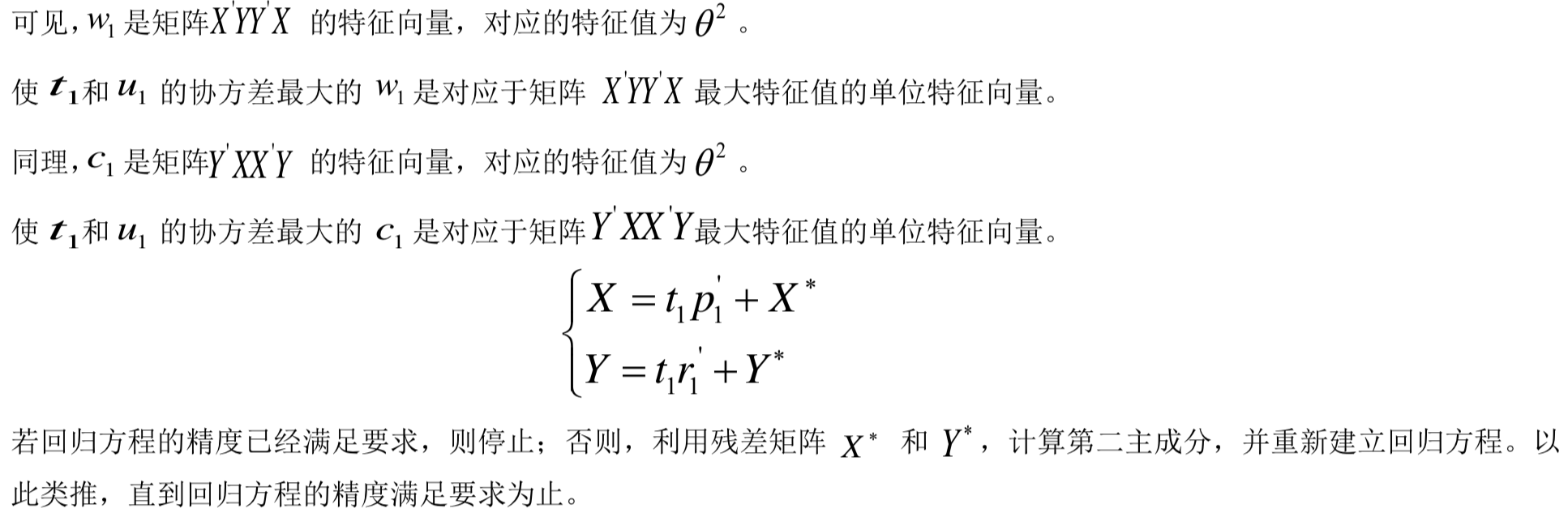## MATLAB实现

### 重点函数解读：

princomp 提取主成分 高级的MATLAB版本使用pca替换了 请查阅matlab文档

COEFF = princomp(X) performs principal components analysis (PCA) on the n-by-p data matrix X, and returns the principal component coefficients, also known as loadings.

https://ww2.mathworks.cn/help/stats/princomp.html?s_tid=doc_ta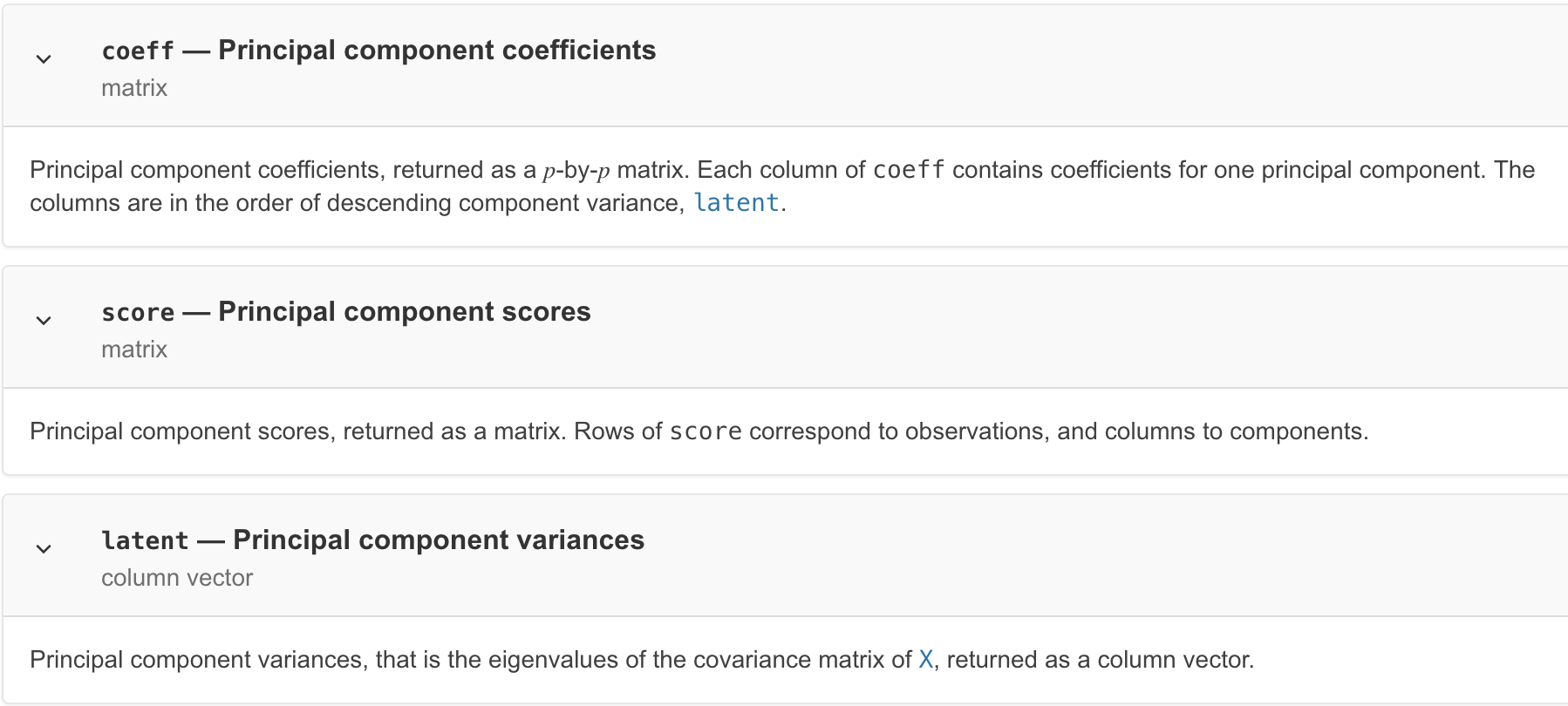regress 多元线性回归

b = regress(y,X) returns a p-by-1 vector b of coefficient estimates for a multilinear regression of the responses in y on the predictors in X.

https://ww2.mathworks.cn/help/stats/regress.html?s_tid=doc_ta

plsregress 偏最小二乘回归

[XL,YL] = plsregress(X,Y,ncomp) computes a partial least-squares (PLS) regression of Y on X, using ncomp PLS components, and returns the predictor and response loadings in XL and YL, respectively.

https://ww2.mathworks.cn/help/stats/plsregress.html?s_tid=doc_ta

### 【例】光谱数据主成分回归分析（PCR）

60个样本，每个样本包含401维度特征的汽油辛烷值光谱测量值。

%% I. 清空环境变量
clear all
clc

%% II. 导入数据
load spectra;

%% III. 随机划分训练集与测试集
temp = randperm(size(NIR, 1));
% temp = 1:60;
%%
% 1. 训练集――50个样本
P_train = NIR(temp(1:50),:);
T_train = octane(temp(1:50),:);
%%
% 2. 测试集――10个样本
P_test = NIR(temp(51:end),:);
T_test = octane(temp(51:end),:);

%% IV. 主成分分析
%%
% 1. 主成分贡献率分析
[PCALoadings,PCAScores,PCAVar] = princomp(NIR);
figure
percent_explained = 100 * PCAVar / sum(PCAVar);
pareto(percent_explained)
xlabel('主成分')
ylabel('贡献率(%)')
title('主成分贡献率')

%%
% 2. 第一主成分vs.第二主成分
[PCALoadings,PCAScores,PCAVar] = princomp(P_train);
figure
plot(PCAScores(:,1),PCAScores(:,2),'r+')
hold on
[PCALoadings_test,PCAScores_test,PCAVar_test] = princomp(P_test);
plot(PCAScores_test(:,1),PCAScores_test(:,2),'o')
xlabel('1st Principal Component')
ylabel('2nd Principal Component')
legend('Training Set','Testing Set','location','best')

%% V. 主成分回归模型
%%
% 1. 创建模型
k = 4;
betaPCR = regress(T_train-mean(T_train),PCAScores(:,1:k));
betaPCR = PCALoadings(:,1:k) * betaPCR;
betaPCR = [mean(T_train)-mean(P_train) * betaPCR;betaPCR];
%%
% 2. 预测拟合
N = size(P_test,1);
T_sim = [ones(N,1) P_test] * betaPCR;

%% VI. 结果分析与绘图
%%
% 1. 相对误差error
error = abs(T_sim - T_test) ./ T_test;
%%
% 2. 决定系数R^2
R2 = (N * sum(T_sim .* T_test) - sum(T_sim) * sum(T_test))^2 / ((N * sum((T_sim).^2) - (sum(T_sim))^2) * (N * sum((T_test).^2) - (sum(T_test))^2));
%%
% 3. 结果对比
result = [T_test T_sim error]

%%
% 4. 绘图
figure
plot(1:N,T_test,'b:*',1:N,T_sim,'r-o')
legend('真实值','预测值','location','best')
xlabel('预测样本')
ylabel('辛烷值')
string = {'测试集辛烷值含量预测结果对比';['R^2=' num2str(R2)]};
title(string)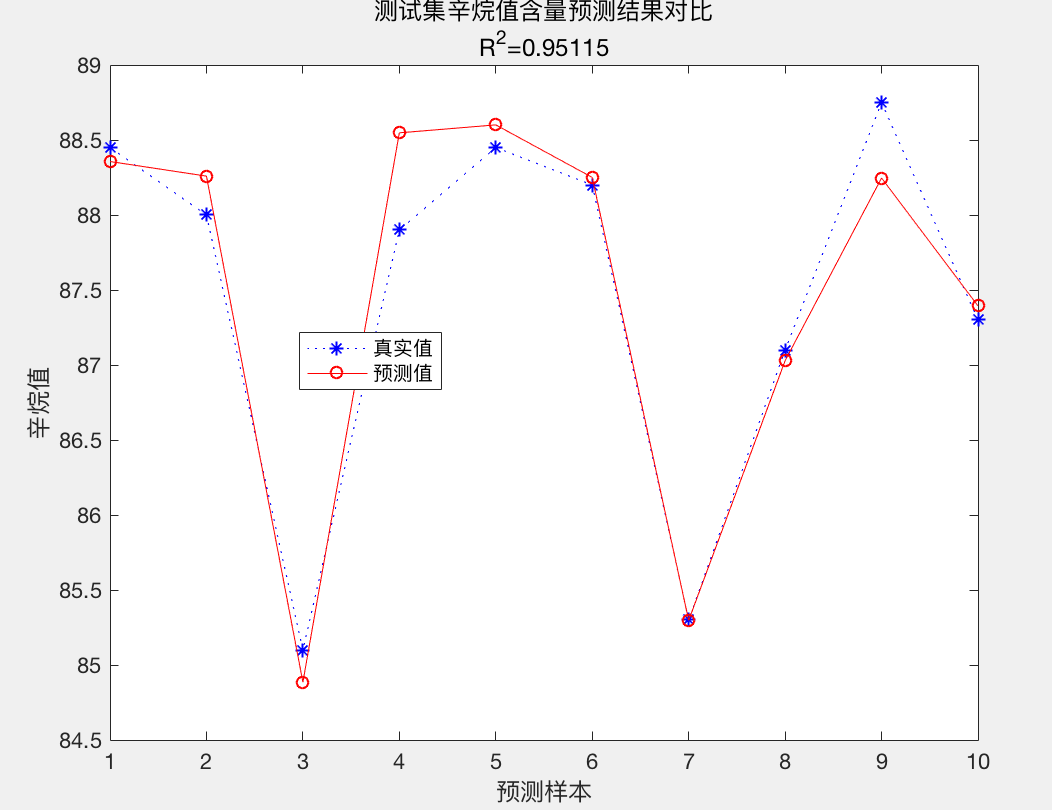（由于代码中训练集和测试集是随机划分的，所以结果可能不同。）

N = size(P_test,1);

T_sim = [ones(N,1) P_test] * betaPCR;

### 【例】偏最小二乘法（PLS）

%% I. 清空环境变量
clear all
clc

%% II. 导入数据
load spectra;

%% III. 随机划分训练集与测试集
temp = randperm(size(NIR, 1));
% temp = 1:60;
%%
% 1. 训练集――50个样本
P_train = NIR(temp(1:50),:);
T_train = octane(temp(1:50),:);
%%
% 2. 测试集――10个样本
P_test = NIR(temp(51:end),:);
T_test = octane(temp(51:end),:);

%% IV. PLS回归模型
%%
% 1. 创建模型
k = 2;
[Xloadings,Yloadings,Xscores,Yscores,betaPLS,PLSPctVar,MSE,stats] = plsregress(P_train,T_train,k);

%%
% 2. 主成分贡献率分析
figure
percent_explained = 100 * PLSPctVar(2,:) / sum(PLSPctVar(2,:));
pareto(percent_explained)
xlabel('主成分')
ylabel('贡献率(%)')
title('主成分贡献率')

%%
% 3. 预测拟合
N = size(P_test,1);
T_sim = [ones(N,1) P_test] * betaPLS;

%% V. 结果分析与绘图
%%
% 1. 相对误差error
error = abs(T_sim - T_test) ./ T_test;
%%
% 2. 决定系数R^2
R2 = (N * sum(T_sim .* T_test) - sum(T_sim) * sum(T_test))^2 / ((N * sum((T_sim).^2) - (sum(T_sim))^2) * (N * sum((T_test).^2) - (sum(T_test))^2));
%%
% 3. 结果对比
result = [T_test T_sim error]

%%
% 4. 绘图
figure
plot(1:N,T_test,'b:*',1:N,T_sim,'r-o')
legend('真实值','预测值','location','best')
xlabel('预测样本')
ylabel('辛烷值')
string = {'测试集辛烷值含量预测结果对比';['R^2=' num2str(R2)]};
title(string)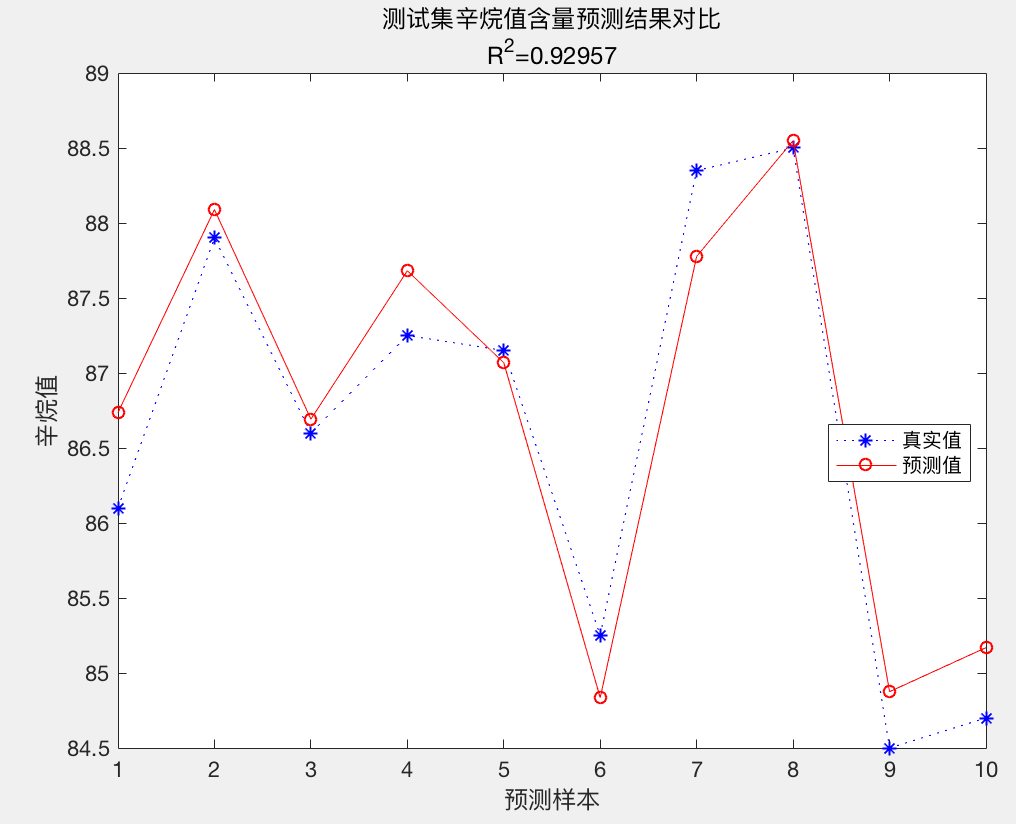# 特征选择的方法

## Filter vs. Wrapper

• Filter：过滤法，按照发散性或者相关性对各个特征进行评分，设定阈值或者待选择阈值的个数，选择特征。
• Wrapper：包装法，根据目标函数（通常是预测效果评分），每次选择若干特征，或者排除若干特征。
• Embedded：嵌入法，先使用某些机器学习的算法和模型进行训练，得到各个特征的权值系数，根据系数从大到小选择特征。类似于Filter方法，但是是通过训练来确定特征的优劣。

Wrapper需要建立学习模型，通过模型的性能进行评价特征的优劣。

Filter无需利用学习模型的性能，即可进行特征选择，主要依赖一些评价准则，如:相关系数、互信息、信息熵等。

## 搜索法

### 随机搜索

• 将N个输入变量用一个长度为N的染色体表示，染色体的每一位代表一个输入变量。

• 每一位的基因取值只能是“1”和“0”两种情况。这一步要注意如何转化，比如如何将二进制编码转化为0或1。

• 如果染色体的某一位值为“1”，表示该位对应的输入变量被选中，参与模型建立。

• 反之，如果染色体的某一位值为“0”，则表示对应的输入变量未被选中，不参与模型建立。

### 启发式搜索

• 前向选择法

- 自下而上的选择方法，又称集合增加法。

- 特征集合初始化为一个空集。

- 每次向特征集合中添加一个输入变量，当新加入的变量致使模型性能更优时，则保留该输入变量，否则不保留。

• 后向选择法

- 自上而下的选择方法，又称集合缩减法

- 特征集合初始化为全部的输入变量

- 每次从特征集合中剔除一个输入变量，当剔除后致使模型性能更优时，则剔除该输入变量，否则保留该输入变量。

• 广义方法

1）一次增加或剔除多个变量

2）区间法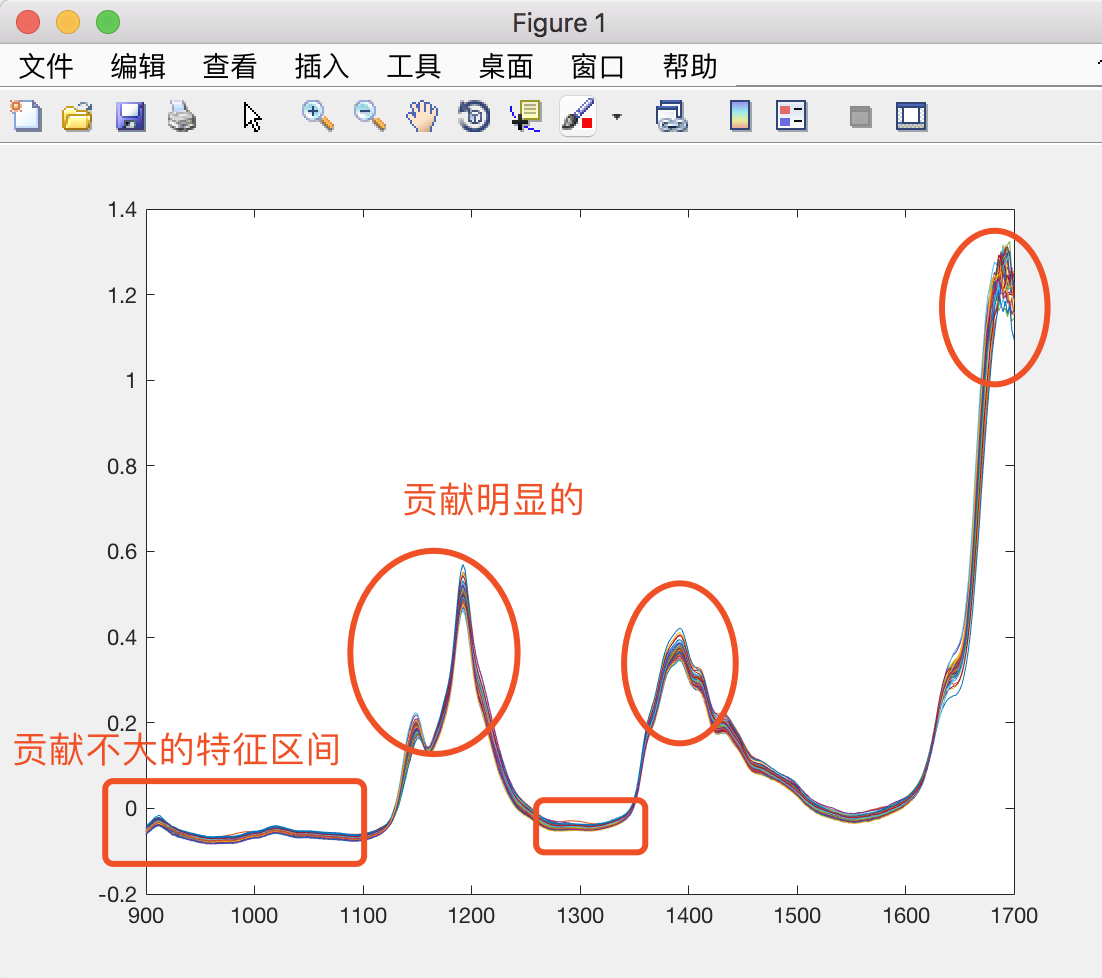## 正则化方法

L1范数、LASSO、稀疏优化……

《深度学习:正则化方法》

# 可视化评价指标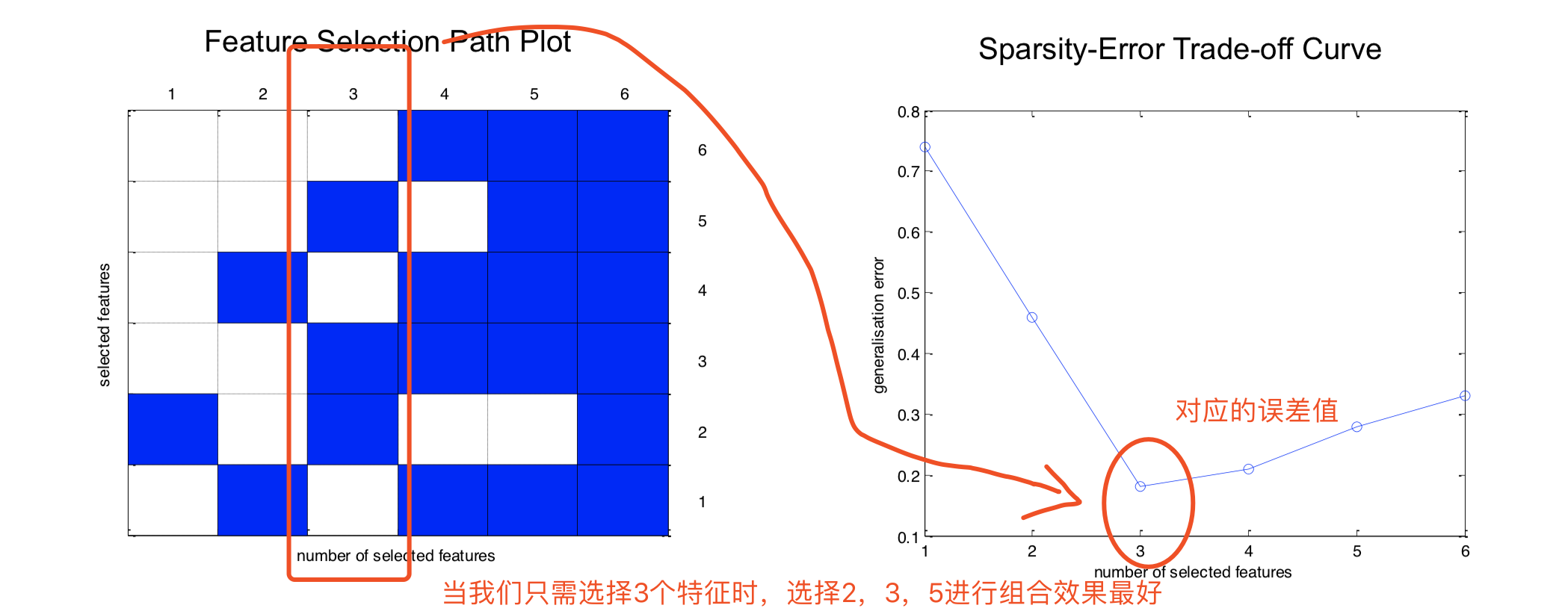07-2011-305083
08-081万+
11-28
11-2816万+
10-317361
05-141万+
01-081万+
09-30396
09-293万+
12-24
10-261800
09-032万+
04-11
10-134604
10-07
05-154360
©️2020 CSDN 皮肤主题: 精致技术 设计师:CSDN官方博客